# Circle Plots with GGPLOT2Pretty circular plots. I’m not sure there is an added benefit for the data analyst herself but if the catch the attention of decision makers than that is added value to me.

Anyway, I think they can look great. However building them with coord_polar(), or the occassionally found coord_radar() in ggplot2 can be a pain. Two main problems I found are that the polar variation bends your paths into circular shapes and radar doesn’t like to work in combination with geom_bin to fill the background.

That’s why I usually work with a ‘hack’ in the cartesian coords system (still in my favourite ggplot2). I wouldn’t really call it a hack, more of a mathematical solution. Map your data and plotting needs so it ends up circular. As an extra benefit, I also find this builds/loads quicker. Important benefit for me, as I make them interactive in Shiny Apps.

I’ve used mtcars data for the example. The plot shows 12 cars from the set with:

• In green background the cylinders. Light, medium and dark green for 4, 6, and 8 cylinders.
• In blue outlining the miles per gallon for each car.
• In grey a made-up range build from the qsec column.

This post is a messy step by step showcase of how to add your desired elements to a circular plot. Many things can probably be improved, please feel free to leave comments. I’d be excited to see extra ‘features’ and cool modifications, please link.

``````library(dplyr)
library(purrr)    # map functions (like lapply)
library(ggplot2)
library(lazyeval) # interp function
``````

I use the first 12 cars and want a column with the rownames.

``````cars <- tbl_df(add_rownames(mtcars, "label")[1:12,]) %>%
mutate(cyl   = factor(cyl),
label = factor(label, levels = unique(label)))
``````

# Plot data mapping

To map the values of any column that I want to plot I created the rotate_data function. It basicly checks how many variables you want to plot (in this case 12, meaning 30Â° for each) and maps sinusoid for the x and y values.

``````# Function

# function requires
rotate_data <- function(data, col, by_col) {
lev <- levels(data[,by_col][])
num <- length(lev)

dir <- rep(seq(((num - 1) * 360 / num), 0, length.out = num))

data\$dir_ <- map_dbl(1:nrow(data), function(x) {dir[match(data[x,by_col][], lev)]})

#col_num <- match("mpg", colnames(cars))
#filter_criteria <- interp(~ which_column == col_num, which_column = as.name(col))

expr <- lazyeval::interp(~x, x = as.name(col))
data <- mutate_(data, .dots = setNames(list(expr), "plotX"))
data <- mutate_(data, .dots = setNames(list(expr), "plotY"))

data <- data %>%
mutate(plotX = round(cos(dir_ * pi / 180) * plotX, 2),
plotY = round(sin(dir_ * pi / 180) * plotY, 2))

data
}
``````

Store mapped data for mapping the mpg variable for all labels.

``````# data points
cars <- rotate_data(cars, "mpg", "label")
``````

I would like to showcase plotting range data so I fake a range of qsec data. Basicly you generate a data frame with multiple values (rows) for qsec on each car (label).

``````# Make up some range data
cars_fake <- bind_rows(cars, mutate(cars, qsec = qsec - 5 * abs(runif(nrow(cars)))))
cars_fake <- rotate_data(cars_fake, "qsec", "label")
``````

Plot the range with geom_polygon, and the mpg values with geom_path and geom_point. Note that ‘close’ the path you can simply add an extra row at the end which is the first row, connecting it to the last.

``````lim <- max(cars\$mpg * 1.1)
# plot each layer with its own data and aesthetics
ggplot() +
geom_polygon(data = cars_fake, aes(y = plotY, x = plotX), fill = "grey70", colour = 'grey70', size = 1, show.legend = FALSE, alpha = 0.8) +
geom_path (data = cars[c(1:nrow(cars),1),], aes(y = plotY, x = plotX), colour = 'steelblue3', size = 1) +
geom_point(data = cars, aes(y = plotY, x = plotX), stat='identity', colour = 'steelblue4', size = 1) +
ylim(-lim, lim) + xlim(-lim, lim) +
theme(
axis.text  = element_blank(),
axis.title = element_blank(),
line       = element_blank(),
rect       = element_blank()
) +
coord_equal()
``````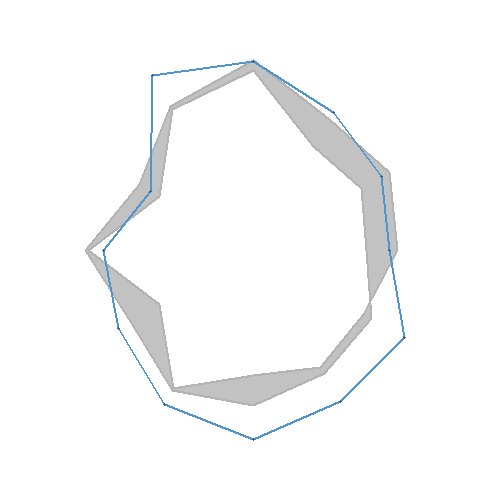I guess the desired grid is build up of radial outward lines with circles. Create x, xend, y, and yend data points to plot segments between.

``````line_length <- max(cars\$mpg * 1.1)
rl <- data_frame(dir = unique(cars\$dir_), l = rep(line_length, length(unique(cars\$dir_)))) %>%
mutate(plotX = cos(dir * pi / 180) * (l),
plotY = sin(dir * pi / 180) * (l))
rl\$xend <- 0
rl\$yend <- 0
``````
``````lim <- max(cars\$mpg * 1.1)
# plot each layer with its own data and aesthetics
ggplot() +
geom_segment(data = rl, aes(x = plotX, xend = xend, y = plotY, yend = yend), colour = "grey50") +
geom_polygon(data = cars_fake, aes(y = plotY, x = plotX), fill = "grey70", colour = 'grey70', size = 1, show.legend = FALSE, alpha = 0.8) +
geom_path   (data = cars[c(1:nrow(cars),1),], aes(y = plotY, x = plotX), colour = 'steelblue3', size = 1) +
geom_point  (data = cars, aes(y = plotY, x = plotX), stat='identity', colour = 'steelblue4', size = 1) +
ylim(-lim, lim) + xlim(-lim, lim) +
theme(
axis.text  = element_blank(),
axis.title = element_blank(),
line       = element_blank(),
rect       = element_blank()
) +
coord_equal()
``````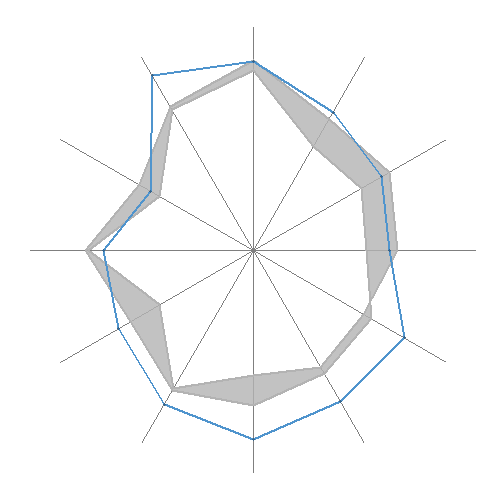# Labels

Add text labels for the variable you rotate around.

``````lb <- rl
lb\$label <- levels(cars\$label)
``````
``````lim <- max(cars\$mpg * 1.1)
# plot each layer with its own data and aesthetics
ggplot() +
geom_segment(data = rl, aes(x = plotX, xend = xend, y = plotY, yend = yend), colour = "grey50") +
geom_polygon(data = cars_fake, aes(y = plotY, x = plotX), fill = "grey70", colour = 'grey70', size = 1, show.legend = FALSE, alpha = 0.8) +
geom_path   (data = cars[c(1:nrow(cars),1),], aes(y = plotY, x = plotX), colour = 'steelblue3', size = 1) +
geom_point  (data = cars, aes(y = plotY, x = plotX), stat='identity', colour = 'steelblue4', size = 1) +
geom_text   (data = lb, aes(x = plotX, y = plotY, label = label), colour = "grey40") +
ylim(-lim, lim) + xlim(-lim, lim) +
theme(
axis.text  = element_blank(),
axis.title = element_blank(),
line       = element_blank(),
rect       = element_blank()
) +
coord_equal()
``````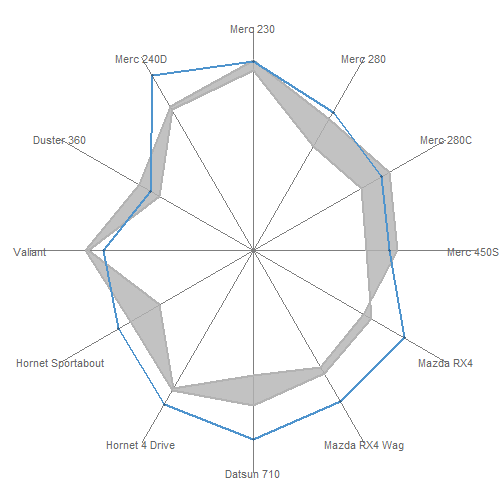# Circle fun

To draw circles I’ll use the circleFun() with fill option. I’ve lost which post to credit for this, so I’ll thank the whole stackoverflow community.

``````circleFun <- function(center=c(0,0), diameter=1, npoints=100, start=0, end=2, filled=TRUE){
tt <- seq(start*pi, end*pi, length.out=npoints)
df <- data.frame(
x = center + diameter / 2 * cos(tt),
y = center + diameter / 2 * sin(tt)
)
if(filled==TRUE) { #add a point at the center so the whole 'pie slice' is filled
df <- rbind(df, center)
}
return(df)
}
``````

# Grid circles and labels

The circle grid lines is build by calling the circleFun several times and storing all the points in a data frame.

``````circlegrid <- data_frame(dia = seq(lim / 4, 2 * lim, lim / 4))
circlegrid <- circlegrid %>%
mutate(data = map(dia, function(x) {
df     <- circleFun(diameter = x, filled = FALSE)
df\$lev <- x
df
}))

plotcircles <- bind_rows(circlegrid\$data)
plotcircles\$lev <- as.factor(plotcircles\$lev)
``````

Circle labels can be added in many ways. But in order to just simply set all axis text and axis labels to element_blank I build a data frame that can be plotted with geom_text.

``````cl <- data_frame(x = as.numeric(levels(plotcircles\$lev)), label = as.character(round(x,1)))
cl <- cl[cl\$x <= max(cars\$mpg * 1.1),]
``````
``````lim <- max(cars\$mpg * 1.1)
# plot each layer with its own data and aesthetics
ggplot() +
geom_segment(data = rl, aes(x = plotX, xend = xend, y = plotY, yend = yend), colour = "grey50") +
geom_path   (data = plotcircles, aes(x = x, y = y, group = lev), colour = "grey50") +
geom_text   (data = cl, aes(x = x, y = 1, label = label), colour = "grey40") +
geom_polygon(data = cars_fake, aes(y = plotY, x = plotX), fill = "grey70", colour = 'grey70', size = 1, show.legend = FALSE, alpha = 0.8) +
geom_path   (data = cars[c(1:nrow(cars),1),], aes(y = plotY, x = plotX), colour = 'steelblue3', size = 1) +
geom_point  (data = cars, aes(y = plotY, x = plotX), stat='identity', colour = 'steelblue4', size = 1) +
geom_text   (data = lb, aes(x = plotX, y = plotY, label = label), colour = "grey40") +
ylim(-lim, lim) + xlim(-lim, lim) +
theme(
axis.text  = element_blank(),
axis.title = element_blank(),
line       = element_blank(),
rect       = element_blank()
) +
coord_equal()
``````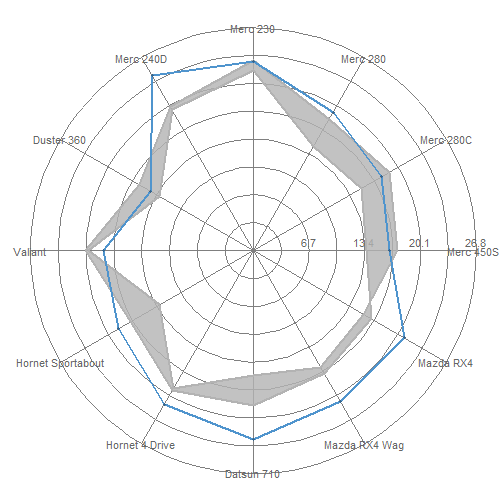# Background

With the circleFun you can also easily build circle section that you can fill with the filled = TRUE argument. There is a little -1/num shift to have the section allign properly. Here you bring forward the factor variable that yuo want to colour in for. You can also change the code to change the ‘height’ of each bar according to a variable of course.

``````# bgdir <- unique(dir) + (unique(dir) - unique(dir))/2
# bgdir <- data_frame(bgdir)
num      <- length(levels(cars\$label))
diameter <- rep(2 * max(cars\$mpg * 1.1), num)
levels   <- rev(cars\$cyl)
start    <- seq(0, (num - 1) * 2 / num, length.out = num) - 1 / num
end      <- seq(2 / num, 2, length.out = num) - 1 / num

bg  <- data_frame(levels   = levels,
diameter = diameter,
start    = start,
end      = end)
bg <- bg %>%
mutate(data = pmap(list(levels, diameter, start, end),
function(x1, x2, x3, x4) {
df     <- circleFun(diameter = x2, start = x3, end = x4, filled = TRUE)
df\$lev <- x1
df
}))

bgdata <- tbl_df(bind_rows(bg\$data))
bgdata\$lev <- as.factor(bgdata\$lev)
``````

# Center Circle

Little detail but you may want to add some center spice.

``````middle <- circleFun(diameter = 1, start=0, end=2, filled = FALSE)
``````
``````lim <- max(cars\$mpg * 1.1)
# plot each layer with its own data and aesthetics
ggplot() +
geom_polygon(data = bgdata, aes(x, y, fill = lev), show.legend = FALSE, alpha = 0.8) +
scale_fill_brewer(palette = "Greens") +
geom_segment(data = rl, aes(x = plotX, xend = xend, y = plotY, yend = yend), colour = "white") +
geom_path   (data = plotcircles, aes(x = x, y = y, group = lev), colour = "white") +
geom_text   (data = cl, aes(x = x, y = 1, label = label), colour = "grey50", size = 3) +
geom_polygon(data = middle, aes(x, y), fill = "steelblue3", colour = "steelblue4") +
geom_polygon(data = cars_fake, aes(y = plotY, x = plotX), fill = "grey70", colour = 'grey70', size = 1, show.legend = FALSE, alpha = 0.8) +
geom_path   (data = cars[c(1:nrow(cars),1),], aes(y = plotY, x = plotX), colour = 'steelblue3', size = 1) +
geom_point  (data = cars, aes(y = plotY, x = plotX), stat='identity', colour = 'steelblue4', size = 1) +
geom_text   (data = lb, aes(x = plotX, y = plotY, label = label), colour = "grey40") +
ylim(-lim, lim) + xlim(-lim, lim) +
theme(
axis.text  = element_blank(),
axis.title = element_blank(),
line       = element_blank(),
rect       = element_blank()
) +
coord_equal()
``````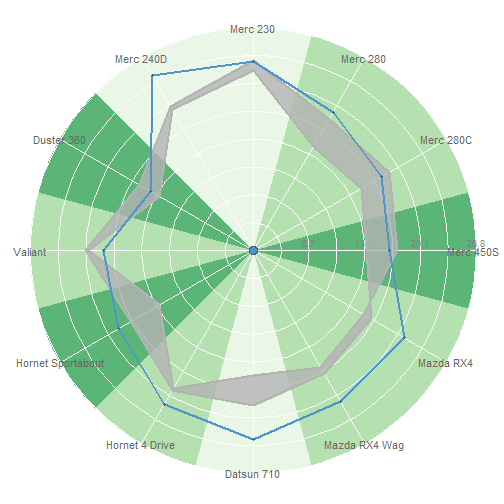### 5 Responses to “Circle Plots with GGPLOT2”

•Benjamin Lee
5 years ago

Hello Jiddu, thank you very much for this post! You saved my life! However, there is one issue when I run your code.

geom_polygon using cars_fake does not provide me sort of ribbon shape but two overlapping polygons completely filled with grey color. I have been using Google for the whole afternoon, trying to solve it. However I failed. So I think I can only ask for help from you.

•Benjamin Lee
5 years ago

I solved it !!!!!! Thank you all the same!! lol

•5 years ago

Hi Benjamin, great that you solved it. Sorry I missed your comment earlier.
Best,
Jiddu

•RV
5 years ago

Hi, I tried to replicate this figure. It is working but, in the first figure, the center part is colored greyed rather than being white as it was shown in the figure (first figure under Plot Data Mapping). Do you know why I am getting this color in the center part? Thanks

•